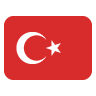4.0Sections

# Image Component

## Genel Bakış

Phalcon\Image is the component that allows you to manipulate image files. Multiple operations can be performed on the same image object.

## Adapters

This component makes use of adapters to encapsulate specific image manipulator programs. The following image manipulator programs are supported:

Class Description
Phalcon\Image\Adapter\Gd Requires the GD PHP extension
Phalcon\Image\Adapter\Imagick Requires the ImageMagick PHP extension

### Factory

Loads an Image Adapter class using `adapter` option.

``````<?php

use Phalcon\Image\Factory;

\$options = [
'width'   => 200,
'height'  => 200,
'file'    => 'upload/test.jpg',
'adapter' => 'imagick',
];

\$image = Factory::load(\$options);
``````

### Kendi Bağdaştırıcılarını Uygulama

The Phalcon\Image\AdapterInterface interface must be implemented in order to create your own image adapters or extend the existing ones.

## Saving and rendering images

Before we begin with the various features of the image component, it’s worth understanding how to save and render these images.

``````<?php

\$image = new \Phalcon\Image\Adapter\Gd('image.jpg');

// ...

// Overwrite the original image
\$image->save();
``````
``````<?php

\$image = new \Phalcon\Image\Adapter\Gd('image.jpg');

// ...

// Save to 'new-image.jpg'
\$image->save('new-image.jpg');
``````

You can also change the format of the image:

``````<?php

\$image = new \Phalcon\Image\Adapter\Gd('image.jpg');

// ...

// Save as a PNG file
\$image->save('image.png');
``````

When saving as a JPEG, you can also specify the quality as the second parameter:

``````<?php

\$image = new \Phalcon\Image\Adapter\Gd('image.jpg');

// ...

// Save as a JPEG with 80% quality
\$image->save('image.jpg', 80);
``````

## Resizing images

There are several modes of resizing:

• `\Phalcon\Image::WIDTH`
• `\Phalcon\Image::HEIGHT`
• `\Phalcon\Image::NONE`
• `\Phalcon\Image::TENSILE`
• `\Phalcon\Image::AUTO`
• `\Phalcon\Image::INVERSE`
• `\Phalcon\Image::PRECISE`

### `\Phalcon\Image::WIDTH`

The height will automatically be generated to keep the proportions the same; if you specify a height, it will be ignored.

``````<?php

\$image = new \Phalcon\Image\Adapter\Gd('image.jpg');

\$image->resize(
300,
null,
\Phalcon\Image::WIDTH
);

\$image->save('resized-image.jpg');
``````

### `\Phalcon\Image::HEIGHT`

The width will automatically be generated to keep the proportions the same; if you specify a width, it will be ignored.

``````<?php

\$image = new \Phalcon\Image\Adapter\Gd('image.jpg');

\$image->resize(
null,
300,
\Phalcon\Image::HEIGHT
);

\$image->save('resized-image.jpg');
``````

### `\Phalcon\Image::NONE`

• The `NONE` constant ignores the original image’s ratio.
• Neither width and height are required.
• If a dimension is not specified, the original dimension will be used.
• If the new proportions differ from the original proportions, the image may be distorted and stretched.
``````<?php

\$image = new \Phalcon\Image\Adapter\Gd('image.jpg');

\$image->resize(
400,
200,
\Phalcon\Image::NONE
);

\$image->save('resized-image.jpg');
``````

### `\Phalcon\Image::TENSILE`

• Similar to the `NONE` constant, the `TENSILE` constant ignores the original image’s ratio.
• Both width and height are required.
• If the new proportions differ from the original proportions, the image may be distorted and stretched.
``````<?php

\$image = new \Phalcon\Image\Adapter\Gd('image.jpg');

\$image->resize(
400,
200,
\Phalcon\Image::TENSILE
);

\$image->save('resized-image.jpg');
``````

## Cropping images

For example, to get a 100px by 100px square from the centre of the image:

``````<?php

\$image = new \Phalcon\Image\Adapter\Gd('image.jpg');

\$width   = 100;
\$height  = 100;
\$offsetX = ((\$image->getWidth() - \$width) / 2);
\$offsetY = ((\$image->getHeight() - \$height) / 2);

\$image->crop(\$width, \$height, \$offsetX, \$offsetY);

\$image->save('cropped-image.jpg');
``````

## Rotating images

``````<?php

\$image = new \Phalcon\Image\Adapter\Gd('image.jpg');

// Rotate an image by 90 degrees clockwise
\$image->rotate(90);

\$image->save('rotated-image.jpg');
``````

## Flipping images

You can flip an image horizontally (using the `\Phalcon\Image::HORIZONTAL` constant) and vertically (using the `\Phalcon\Image::VERTICAL` constant):

``````<?php

\$image = new \Phalcon\Image\Adapter\Gd('image.jpg');

// Flip an image horizontally
\$image->flip(
\Phalcon\Image::HORIZONTAL
);

\$image->save('flipped-image.jpg');
``````

## Sharpening images

The `sharpen()` method takes a single parameter - an integer between 0 (no effect) and 100 (very sharp):

``````<?php

\$image = new \Phalcon\Image\Adapter\Gd('image.jpg');

\$image->sharpen(50);

\$image->save('sharpened-image.jpg');
``````

## Adding watermarks to images

``````<?php

\$image = new \Phalcon\Image\Adapter\Gd('image.jpg');

\$watermark = new \Phalcon\Image\Adapter\Gd('me.jpg');

// Put the watermark in the top left corner
\$offsetX = 10;
\$offsetY = 10;

\$opacity = 70;

\$image->watermark(
\$watermark,
\$offsetX,
\$offsetY,
\$opacity
);

\$image->save('watermarked-image.jpg');
``````

Of course, you can also manipulate the watermarked image before applying it to the main image:

``````<?php

\$image = new \Phalcon\Image\Adapter\Gd('image.jpg');

\$watermark = new \Phalcon\Image\Adapter\Gd('me.jpg');

\$watermark->resize(100, 100);
\$watermark->rotate(90);
\$watermark->sharpen(5);

// Put the watermark in the bottom right corner with a 10px margin
\$offsetX = (\$image->getWidth() - \$watermark->getWidth() - 10);
\$offsetY = (\$image->getHeight() - \$watermark->getHeight() - 10);

\$opacity = 70;

\$image->watermark(
\$watermark,
\$offsetX,
\$offsetY,
\$opacity
);

\$image->save('watermarked-image.jpg');
``````

## Blurring images

The `blur()` method takes a single parameter - an integer between 0 (no effect) and 100 (very blurry):

``````<?php

\$image = new \Phalcon\Image\Adapter\Gd('image.jpg');

\$image->blur(50);

\$image->save('blurred-image.jpg');
``````

## Pixelating images

The `pixelate()` method takes a single parameter - the higher the integer, the more pixelated the image becomes:

``````<?php

\$image = new \Phalcon\Image\Adapter\Gd('image.jpg');

\$image->pixelate(10);

\$image->save('pixelated-image.jpg');
``````